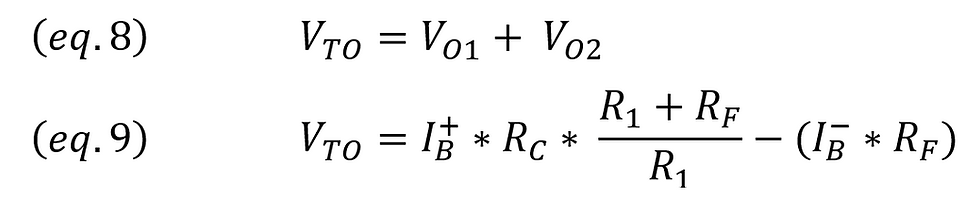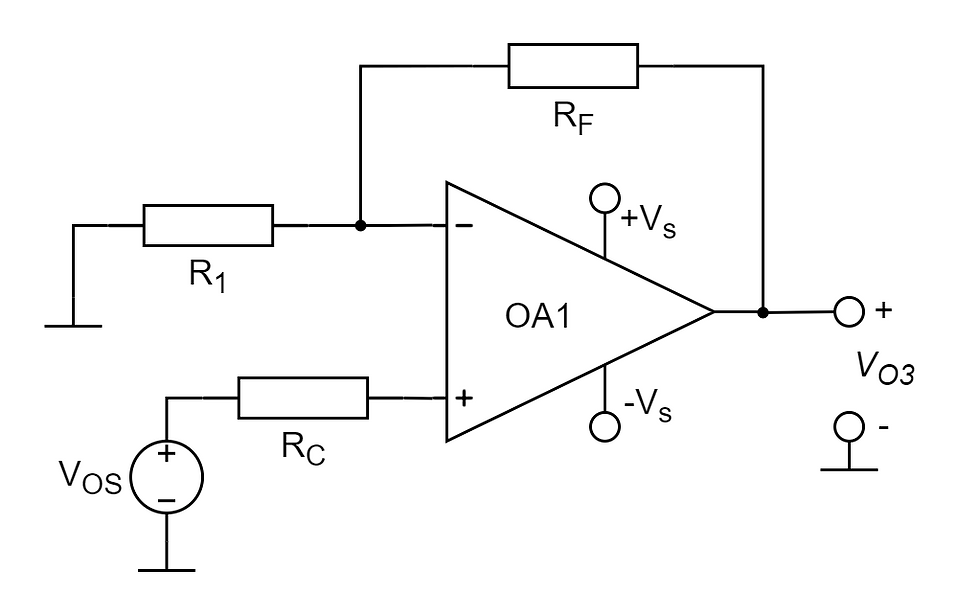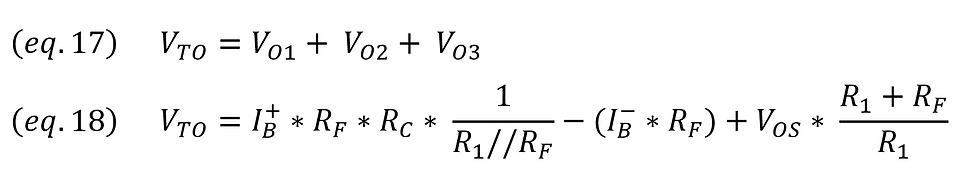top of page
Search

# Opamp Bias Current, Offset Current and Offset VoltageInput bias and offset currents of opamps

Below are excerpts from technical documents of two different general-purpose op-amps as examples.Figure 1: (A) Technical document excerpts for the AD8538 and (B) LM747 op-amps.

To investigate the effect of Ios, Vos, and Ib parameters indicated in the technical documents on the op-amp output, a sample op-amp circuit with negative feedback is provided below.Figure 2: Op-amp circuit with negative feedback.

## Bias Current & Offset Current (exc: Opamp Offset Voltage)

To only observe the effect of bias currents in the sample feedback op-amp circuit in Figure 2, the input voltage is changed to 0V. By showing the input bias currents on the circuit diagram, the schema in Figure 3(A) is obtained.Figure 3: (A) Circuit obtained when the input voltage of the example op-amp circuit is selected as 0V and the input bias currents are shown on the circuit diagram. (B) Simplified circuit.

The terms IB+ and IB− and their equivalents for the "Input Bias Current" and "Input Offset Current" parameters mentioned in the technical documents are represented in equations eq.1 and eq.2.Equations for opamp input bias and offset currents

In the obtained circuit diagram, the IB+ current will not cause a voltage difference at the op-amp input. In this case, the voltage at the V- point will be 0V, and since no current will pass through the R1 resistor, all of the IB- current will pass through the RF resistor. The simplified version of the sample circuit is shown in Figure 3(B).

The voltage observed at the output of the opamp in Figure 3(B) will be represented by the equation eq.3.Equations for opamp input bias and offset currents

## Compensation Resistance

If an RC compensation resistor is added to the sample feedback op-amp circuit, the schema in Figure 4 is obtained.Figure 4: Circuit diagram obtained by adding the RC compensation resistor.

In the feedback op-amp circuit in Figure 4, to only observe the effect of bias currents, the input voltage is changed to 0V. By showing the input bias currents on the circuit diagram, the schema in Figure 5 is obtained.Figure 5: The circuit obtained when the input voltage of the sample op-amp circuit is selected as 0V and the input bias currents are shown on the circuit diagram.

In order to calculate the voltage to be seen at the opamp output in the diagram in Figure 5, the effect of each of the IB+ and IB- current sources on the opamp output is calculated separately.Figure 6: (A) Demonstration of the state where only the IB+ current source is connected. (B) Indication of the state where only the IB- current source is connected.

From the circuit diagram in Figure 6 (A), (eq.4) and (eq.5) are obtained.Equations for calculating bias and offset voltage

From the circuit diagram in Figure 6 (B), (eq.6) and (eq.7) are obtained.Equations for calculating bias and offset voltage

If the equations for the circuit diagram in Figure 5 are summed up, the result (eq.9) is reached by considering (eq.8).Equations for calculating bias and offset voltage

In order to better see the effect of the RC resistor, the (eq.10) and (eq.11) equations have been adjusted.Equations for calculating bias and offset voltage

At this point, if the RC resistance is equal to the parallel sum of the R1 and RF resistors as shown in the equation (eq.12), the equation (eq.14) is reached.Equations for calculating bias and offset voltage

When we use the (eq.2) equation in the (eq.14) equation, we finally get the (eq.15) equation.Equations for calculating bias and offset voltage

Consequently, the RC compensation resistor is implemented in circuit diagrams with the intent of maintaining lower voltage discrepancies at the output, especially in opamps where the "Input Bias Current" value significantly surpasses the "Input Offset Current" value. Most of the time, the RC resistor is selected to match the parallel summation of the R1 and RF resistors.

## Opamp Offset Voltage

To analyze the influence of bias currents and offset voltage on the output of the opamp within the example negative feedback opamp configuration depicted in Figure 4, the input voltage was adjusted to 0V. Figure 7 presents the derived diagram, showcasing both the input bias currents and the offset voltage on the circuit schematic.Figure 8: Display of the situation where only the VOS voltage source is connected.

The equation (eq.16) is obtained from the circuit diagram in Figure 8.Equations for calculating bias and offset voltage

If the equations for the circuit diagram in Figure 7 are summed up (eq.17), the result (eq.18) is reached.Equations for calculating bias and offset voltage

If the RC resistor is set equal to the parallel combination of the R1 and RF resistors, as indicated in equation eq.12, and by following analogous steps, then for the opamp circuit in Figure 4 with an input voltage of 0V, the equation eq.19 represents the total offset voltage observed at the output.Final obtained equation